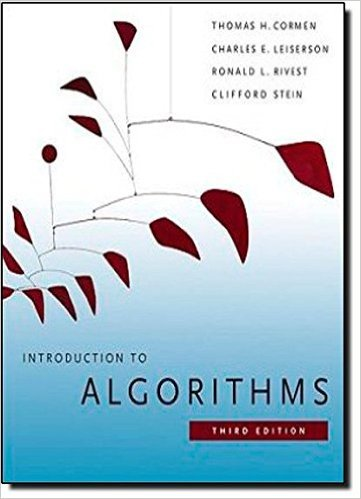×
Get Full Access to Introduction To Algorithms - 3 Edition - Chapter 6 - Problem 6-3
Get Full Access to Introduction To Algorithms - 3 Edition - Chapter 6 - Problem 6-3

×

# Young tableaus An m n Young tableau is an m n matrix suchISBN: 9780262033848 130

## Solution for problem 6-3 Chapter 6

Introduction to Algorithms | 3rd Edition

• Textbook Solutions
• 2901 Step-by-step solutions solved by professors and subject experts
• Get 24/7 help from StudySoup virtual teaching assistantsIntroduction to Algorithms | 3rd Edition

4 5 1 243 Reviews
13
5
Problem 6-3

Young tableaus An m n Young tableau is an m n matrix such that the entries of each row are in sorted order from left to right and the entries of each column are in sorted order from top to bottom. Some of the entries of a Young tableau may be 1, which we treat as nonexistent elements. Thus, a Young tableau can be used to hold r mn finite numbers. a. Draw a 4 4 Young tableau containing the elements f9; 16; 3; 2; 4; 8; 5; 14; 12g. b. Argue that an m n Young tableau Y is empty if Y 1; 1 D 1. Argue that Y is full (contains mn elements) if Y m; n < 1. 168 Chapter 6 Heapsort c. Give an algorithm to implement EXTRACT-MIN on a nonempty m n Young tableau that runs in O.m C n/ time. Your algorithm should use a recursive subroutine that solves an m n problem by recursively solving either an .m 1/ n or an m .n 1/ subproblem. (Hint: Think about MAXHEAPIFY.) Define T .p/, where p D m C n, to be the maximum running time of EXTRACT-MIN on any m n Young tableau. Give and solve a recurrence for T .p/ that yields the O.m C n/ time bound. d. Show how to insert a new element into a nonfull m n Young tableau in O.m C n/ time. e. Using no other sorting method as a subroutine, show how to use an n n Young tableau to sort n2 numbers in O.n3/ time. f. Give an O.m C n/-time algorithm to determine whether a given number is stored in a given m n Young tableau.

Step-by-Step Solution:
Step 1 of 3

L11 - 2 Def. The line y = L is called a horizontal asymptote of the graph of f(x)f i How many horizontal asymptotes can a graph have Consider the following functions: x ex. f(x)= x ex. f(x)=1 − e ✻ ✻ ✛ ✲ ✛ ✲ ❄ ❄ −1 ex. f(x)=t n (x) ✻ ✛ ✲ ❄ −1 −1 x→∞m tan (x)=

Step 2 of 3

Step 3 of 3

##### ISBN: 9780262033848

Since the solution to 6-3 from 6 chapter was answered, more than 524 students have viewed the full step-by-step answer. The answer to “Young tableaus An m n Young tableau is an m n matrix such that the entries of each row are in sorted order from left to right and the entries of each column are in sorted order from top to bottom. Some of the entries of a Young tableau may be 1, which we treat as nonexistent elements. Thus, a Young tableau can be used to hold r mn finite numbers. a. Draw a 4 4 Young tableau containing the elements f9; 16; 3; 2; 4; 8; 5; 14; 12g. b. Argue that an m n Young tableau Y is empty if Y 1; 1 D 1. Argue that Y is full (contains mn elements) if Y m; n < 1. 168 Chapter 6 Heapsort c. Give an algorithm to implement EXTRACT-MIN on a nonempty m n Young tableau that runs in O.m C n/ time. Your algorithm should use a recursive subroutine that solves an m n problem by recursively solving either an .m 1/ n or an m .n 1/ subproblem. (Hint: Think about MAXHEAPIFY.) Define T .p/, where p D m C n, to be the maximum running time of EXTRACT-MIN on any m n Young tableau. Give and solve a recurrence for T .p/ that yields the O.m C n/ time bound. d. Show how to insert a new element into a nonfull m n Young tableau in O.m C n/ time. e. Using no other sorting method as a subroutine, show how to use an n n Young tableau to sort n2 numbers in O.n3/ time. f. Give an O.m C n/-time algorithm to determine whether a given number is stored in a given m n Young tableau.” is broken down into a number of easy to follow steps, and 283 words. The full step-by-step solution to problem: 6-3 from chapter: 6 was answered by , our top Engineering and Tech solution expert on 11/10/17, 05:55PM. This textbook survival guide was created for the textbook: Introduction to Algorithms, edition: 3. Introduction to Algorithms was written by and is associated to the ISBN: 9780262033848. This full solution covers the following key subjects: young, tableau, elements, give, entries. This expansive textbook survival guide covers 35 chapters, and 151 solutions.

Unlock Textbook Solution